3.6 An Example of REM and LSDV

In this rather lengthy example to show the relationships between the REM and LSDV model we explore several different transformations of the data and model specifications. In a set of examples we will use all fifty observations. In two sets of results we will use the five observations on the individual means and the ten observations on the time means.
The following tables show the means of the variables in column two. The other columns are standard deviation, minimum and maximum. The original data is from problem set 2.

Group means for the independent variable.
1             2             3             4             5
ROW   1   10.0000       30.6060       11.5886       41.6700       3.78000
ROW   2   10.0000       29.7270       8.92409       47.7000       18.6600
ROW   3   10.0000       25.9480       8.81008       41.4200       12.5600
ROW   4   10.0000       28.4260       9.72321       42.2500       14.8000
ROW   5   10.0000       24.3030       12.4954       41.4400       5.75000

Group means for the dependent variable
1             2             3             4             5
ROW   1   10.0000       47.8690       12.9291       65.2700       21.7100
ROW   2   10.0000       37.2290       9.54634       49.3900       16.2100
ROW   3   10.0000       38.1330       10.5279       51.3700       14.5100
ROW   4   10.0000       40.7300       14.6780       56.1200       9.31000
ROW   5   10.0000       34.5160       12.4180       47.9600       14.8300

Time means for the independent variable
1             2             3             4             5
ROW   1   5.00000       36.9220       4.07979       41.8600       32.5200
ROW   2   5.00000       25.5600       7.09338       35.3200       18.5200
ROW   3   5.00000       22.2480       11.2742       34.2100       3.78000
ROW   4   5.00000       21.7460       8.68052       35.3400       14.0200
ROW   5   5.00000       28.0860       5.30027       35.1300       20.8300
ROW   6   5.00000       27.3200       11.5646       39.1900       10.3700
ROW   7   5.00000       25.3820       18.2429       47.7000       5.75000
ROW   8   5.00000       21.6140       7.45824       30.7100       12.5600
ROW   9   5.00000       30.7500       5.37027       36.8600       23.7000
ROW  10   5.00000       38.3920       6.26952       42.2500       27.3200

Time means for the dependent variable
1             2             3             4             5
ROW   1   5.00000       50.1320       6.60087       60.5900       42.7900
ROW   2   5.00000       41.3000       6.60381       50.0400       33.8200
ROW   3   5.00000       20.4060       11.9642       40.2900       9.31000
ROW   4   5.00000       39.4320       8.72853       49.9100       25.6100
ROW   5   5.00000       34.6540       7.38297       45.8800       28.2400
ROW   6   5.00000       42.3960       13.0091       53.5500       20.7900
ROW   7   5.00000       45.6340       18.8894       65.2700       14.8300
ROW   8   5.00000       35.2340       10.1048       45.0000       22.8000
ROW   9   5.00000       38.1080       5.73346       44.6200       31.8600
ROW  10   5.00000       49.6580       7.52705       59.1800       42.6200

Grand MEAN of the dependent variable Y = 39.6954

Grand mean of the independent variable X = 27.8020

The following table presents the results using OLS, before anything has been done to the data.

Ordinary    least squares regression.     Dep. Variable     =  Y
Observations       =             50       Weights           =  ONE
Mean of LHS        =  0.3969540E+02       Std.Dev of LHS    =  0.1252044E+02
StdDev of residuals=  0.1067064E+02       Sum of squares    =  0.5465407E+04
Rşsquared          =  0.2884798E+00       Adjusted Rşsquared=  0.2736564E+00
F[  1,    48]      =  0.1946119E+02
Logşlikelihood     = ş0.1883012E+03       Restr.(▀=0) Logşl = ş0.1968100E+03
Amemiya Pr. Criter.=  0.7612048E+01       Akaike Info.Crit. =  0.1184171E+03
ANOVA  Source         Variation     Degrees of Freedom       Mean Square
Regression     0.2215902E+04            1.              0.2215902E+04
Residual       0.5465407E+04           48.              0.1138626E+03
Total          0.7681309E+04           49.              0.1567614E+03
DurbinşWatson stat.=   2.1803752          Autocorrelation   =  ş0.0901876
Variable  Coefficient  Std. Error   tşratio Prob|t|x   Mean of X  Std.Dev.of X
şşşşşşşşşşşşşşşşşşşşşşşşşşşşşşşşşşşşşşşşşş

73;şşşşşşşşşşşşşşşşşşşşşşşşşşşşşşşşşşşş
Constant   21.470        4.398        4.881  0.00001
X1        0.65554       0.1486        4.411  0.00006   27.802        10.258

The following table presents the results for a model in which the data has been transformed by subtracting off the appropriate group mean and the grand mean from each data point.

Ordinary    least squares regression.     Dep. Variable     =  YIND
Observations       =             50       Weights           =  ONE
Mean of LHS        = ş0.3969540E+02       Std.Dev of LHS    =  0.1164879E+02
StdDev of residuals=  0.1002805E+02       Sum of squares    =  0.4826970E+04
Rşsquared          =  0.2740333E+00       Adjusted Rşsquared=  0.2589090E+00
F[  1,    48]      =  0.1811873E+02
Logşlikelihood     = ş0.1851957E+03       Restr.(▀=0) Logşl = ş0.1932020E+03
Amemiya Pr. Criter.=  0.7487828E+01       Akaike Info.Crit. =  0.1045843E+03
ANOVA  Source         Variation     Degrees of Freedom       Mean Square
Regression     0.1822054E+04            1.              0.1822054E+04
Residual       0.4826970E+04           48.              0.1005619E+03
Total          0.6649024E+04           49.              0.1356944E+03
DurbinşWatson stat.=   2.4034218          Autocorrelation   =  ş0.2017109
Variable  Coefficient  Std. Error   tşratio Prob|t|x   Mean of X  Std.Dev.of X
şşşşşşşşşşşşşşşşşşşşşşşşşşşşşşşşşşşşşşşşşş

73;şşşşşşşşşşşşşşşşşşşşşşşşşşşşşşşşşşşş
Constant  ş22.707        4.235       ş5.361  0.00000
XIND      0.61104       0.1436        4.257  0.00010  ş27.802        9.9796

In this table we present the results for the original data, but with the group dummies included. As we found in the lecture notes, the slope coefficients are the same as those in the ANOVA model.

Ordinary    least squares regression.     Dep. Variable     =  Y
Observations       =             50       Weights           =  ONE
Mean of LHS        =  0.3969540E+02       Std.Dev of LHS    =  0.1252044E+02
StdDev of residuals=  0.1047396E+02       Sum of squares    =  0.4826970E+04
Rşsquared          =  0.3715954E+00       Adjusted Rşsquared=  0.3001858E+00
F[  5,    44]      =  0.5203717E+01
Logşlikelihood     = ş0.1851957E+03       Restr.(▀=0) Logşl = ş0.1968100E+03
Amemiya Pr. Criter.=  0.7647828E+01       Akaike Info.Crit. =  0.1228683E+03

ANOVA  Source         Variation     Degrees of Freedom       Mean Square
Regression     0.2854339E+04            5.              0.5708678E+03
Residual       0.4826970E+04           44.              0.1097039E+03
Total          0.7681309E+04           49.              0.1567614E+03
DurbinşWatson stat.=   2.4034217          Autocorrelation   =  ş0.2017109
Variable  Coefficient  Std. Error   tşratio Prob|t|x   Mean of X  Std.Dev.of X
şşşşşşşşşşşşşşşşşşşşşşşşşşşşşşşşşşşşşşşşşş

73;şşşşşşşşşşşşşşşşşşşşşşşşşşşşşşşşşşşş
X1        0.61104       0.1499        4.075  0.00019   27.802        10.258
I1         29.168        5.659        5.154  0.00001  0.20000       0.40406
I2         19.065        5.553        3.433  0.00131  0.20000       0.40406
I3         22.278        5.109        4.360  0.00008  0.20000       0.40406
I4         23.361        5.398        4.328  0.00009  0.20000       0.40406
I5         19.666        4.924        3.994  0.00024  0.20000       0.40406

A graphical view of the individual effects model provides some insight into the gains from this approach.

In the following table we present the results for a regression in which the group mean appears in each period and then grand means have been subtracted.

Ordinary    least squares regression.     Dep. Variable     =  YIND
Observations       =             50       Weights           =  ONE
Mean of LHS        = ş0.7629395Eş06       Std.Dev of LHS    =  0.4589886E+01
StdDev of residuals=  0.3057628E+01       Sum of squares    =  0.4581054E+03
Rşsquared          =  0.5562222E+00       Adjusted Rşsquared=  0.5562222E+00
F[  1,    49]      =  0.6141561E+02
Logşlikelihood     = ş0.1263238E+03       Restr.(▀=0) Logşl = ş0.1466346E+03
Amemiya Pr. Criter.=  0.5092953E+01       Akaike Info.Crit. =  0.9536071E+01
ANOVA  Source         Variation     Degrees of Freedom       Mean Square
Regression     0.5741801E+03            1.              0.5741801E+03
Residual       0.4581054E+03           49.              0.9349090E+01
Total          0.1032285E+04           49.              0.2106705E+02
DurbinşWatson stat.=   0.2820563          Autocorrelation   =   0.8589718
Variable  Coefficient  Std. Error   tşratio Prob|t|x   Mean of X  Std.Dev.of X
şşşşşşşşşşşşşşşşşşşşşşşşşşşşşşşşşşşşşşşşşş

73;şşşşşşşşşşşşşşşşşşşşşşşşşşşşşşşşşşşş
XIND       1.4414       0.1839        7.837  0.00000 ş0.38147Eş06    2.3749

The following table is based on the five observations we have remaining after we average across time for each individual. Not surprisingly, the slope coefficient looks just like the table above it. If you will look at section 3.3 Estimation in the lecture notes, you will see that this equivalence was pointed out in step 1.a

Ordinary    least squares regression.     Dep. Variable     =  Y
Observations       =              5       Weights           =  ONE
Mean of LHS        =  0.3969540E+02       Std.Dev of LHS    =  0.5080072E+01
StdDev of residuals=  0.3907707E+01       Sum of squares    =  0.4581052E+02
Rşsquared          =  0.5562224E+00       Adjusted Rşsquared=  0.4082965E+00
F[  1,     3]      =  0.3760143E+01
Logşlikelihood     = ş0.1263238E+02       Restr.(▀=0) Logşl = ş0.1466346E+02
Amemiya Pr. Criter.=  0.5852953E+01       Akaike Info.Crit. =  0.2137824E+02
ANOVA  Source         Variation     Degrees of Freedom       Mean Square
Regression     0.5741803E+02            1.              0.5741803E+02
Residual       0.4581052E+02            3.              0.1527017E+02
Total          0.1032285E+03            4.              0.2580714E+02
DurbinşWatson stat.=   2.8205634          Autocorrelation   =  ş0.4102817
Variable  Coefficient  Std. Error   tşratio Prob|t|x   Mean of X  Std.Dev.of X
şşşşşşşşşşşşşşşşşşşşşşşşşşşşşşşşşşşşşşşşşş

73;şşşşşşşşşşşşşşşşşşşşşşşşşşşşşşşşşşşş
Constant ş0.37733        20.74       ş0.018  0.98663
X          1.4414       0.7433        1.939  0.14784   27.802        2.6286

In the table which follows, we have subtracted the appropriate time mean and grand mean from each observation.

Ordinary    least squares regression.     Dep. Variable     =  YTIM
Observations       =             50       Weights           =  ONE
Mean of LHS        = ş0.3969540E+02       Std.Dev of LHS    =  0.9383881E+01
StdDev of residuals=  0.8391422E+01       Sum of squares    =  0.3379966E+04
Rşsquared          =  0.2166583E+00       Adjusted Rşsquared=  0.2003386E+00
F[  1,    48]      =  0.1327594E+02
Logşlikelihood     = ş0.1762869E+03       Restr.(▀=0) Logşl = ş0.1823915E+03
Amemiya Pr. Criter.=  0.7131475E+01       Akaike Info.Crit. =  0.7323260E+02
ANOVA  Source         Variation     Degrees of Freedom       Mean Square
Regression     0.9348379E+03            1.              0.9348379E+03
Residual       0.3379966E+04           48.              0.7041596E+02
Total          0.4314804E+04           49.              0.8805722E+02
DurbinşWatson stat.=   1.9365728          Autocorrelation   =   0.0317136
Variable  Coefficient  Std. Error   tşratio Prob|t|x   Mean of X  Std.Dev.of X
şşşşşşşşşşşşşşşşşşşşşşşşşşşşşşşşşşşşşşşşşş

73;şşşşşşşşşşşşşşşşşşşşşşşşşşşşşşşşşşşş
Constant  ş25.431        4.091       ş6.217  0.00000
XTIM      0.51307       0.1408        3.644  0.00066  ş27.802        8.5133

The following table presents the results for the model with time dummies used with the original data. Once again we see that the ANOVA and LSDV models are the same.

Ordinary    least squares regression.     Dep. Variable     =  Y
Observations       =             50       Weights           =  ONE
Mean of LHS        =  0.3969540E+02       Std.Dev of LHS    =  0.1252044E+02
StdDev of residuals=  0.9309447E+01       Sum of squares    =  0.3379966E+04
Rşsquared          =  0.5599752E+00       Adjusted Rşsquared=  0.4471484E+00
F[ 10,    39]      =  0.4963137E+01
Logşlikelihood     = ş0.1762869E+03       Restr.(▀=0) Logşl = ş0.1968100E+03
Amemiya Pr. Criter.=  0.7491475E+01       Akaike Info.Crit. =  0.1057323E+03
ANOVA  Source         Variation     Degrees of Freedom       Mean Square
Regression     0.4301343E+04           10.              0.4301343E+03
Residual       0.3379966E+04           39.              0.8666580E+02
Total          0.7681309E+04           49.              0.1567614E+03
DurbinşWatson stat.=   1.9365728          Autocorrelation   =   0.0317136
Variable  Coefficient  Std. Error   tşratio Prob|t|x   Mean of X  Std.Dev.of X
şşşşşşşşşşşşşşşşşşşşşşşşşşşşşşşşşşşşşşşşşş

73;şşşşşşşşşşşşşşşşşşşşşşşşşşşşşşşşşşşş
X1        0.51307       0.1562        3.284  0.00216   27.802        10.258
T1         31.189        7.113        4.384  0.00009  0.10000       0.30305
T2         28.186        5.769        4.886  0.00002  0.10000       0.30305
T3         8.9913        5.423        1.658  0.10536  0.10000       0.30305
T4         28.275        5.373        5.262  0.00001  0.10000       0.30305
T5         20.244        6.048        3.347  0.00182  0.10000       0.30305
T6         28.379        5.962        4.760  0.00003  0.10000       0.30305
T7         32.611        5.749        5.672  0.00000  0.10000       0.30305
T8         24.145        5.360        4.504  0.00006  0.10000       0.30305
T9         22.331        6.357        3.513  0.00114  0.10000       0.30305
T10        29.960        7.301        4.104  0.00020  0.10000       0.30305

A graphical view of the time fixed effects model clarifies the issues a bit.

In the following table, the time mean is used for each individual, then the grand mean is subtracted.

Ordinary    least squares regression.     Dep. Variable     =  YTIM
Observations       =             50       Weights           =  ONE
Mean of LHS        = ş0.1907349Eş06       Std.Dev of LHS    =  0.8288798E+01
StdDev of residuals=  0.6150913E+01       Sum of squares    =  0.1853853E+04
Rşsquared          =  0.4493242E+00       Adjusted Rşsquared=  0.4493242E+00
F[  1,    49]      =  0.3998158E+02
Logşlikelihood     = ş0.1612719E+03       Restr.(▀=0) Logşl = ş0.1761871E+03
Amemiya Pr. Criter.=  0.6490875E+01       Akaike Info.Crit. =  0.3859040E+02
ANOVA  Source         Variation     Degrees of Freedom       Mean Square
Regression     0.1512652E+04            1.              0.1512652E+04
Residual       0.1853853E+04           49.              0.3783373E+02
Total          0.3366505E+04           49.              0.6870418E+02
DurbinşWatson stat.=   2.7435652          Autocorrelation   =  ş0.3717826
Variable  Coefficient  Std. Error   tşratio Prob|t|x   Mean of X  Std.Dev.of X
şşşşşşşşşşşşşşşşşşşşşşşşşşşşşşşşşşşşşşşşşş

73;şşşşşşşşşşşşşşşşşşşşşşşşşşşşşşşşşşşş
XTIM      0.97077       0.1535        6.323  0.00000 ş0.19073Eş06    5.7234

The table which follows is based on the ten observations which result when we average across people. The slope coefficient differs a bit due to rounding errors.

Ordinary    least squares regression.     Dep. Variable     =  Y
Observations       =             10       Weights           =  ONE
Mean of LHS        =  0.3969460E+02       Std.Dev of LHS    =  0.8650567E+01
StdDev of residuals=  0.6809286E+01       Sum of squares    =  0.3709310E+03
Rşsquared          =  0.4492411E+00       Adjusted Rşsquared=  0.3803962E+00
F[  1,     8]      =  0.6525412E+01
Logşlikelihood     = ş0.3225654E+02       Restr.(▀=0) Logşl = ş0.3523883E+02
Amemiya Pr. Criter.=  0.6851308E+01       Akaike Info.Crit. =  0.5563965E+02

ANOVA  Source         Variation     Degrees of Freedom       Mean Square
Regression     0.3025597E+03            1.              0.3025597E+03
Residual       0.3709310E+03            8.              0.4636638E+02
Total          0.6734907E+03            9.              0.7483230E+02
DurbinşWatson stat.=   2.7358262          Autocorrelation   =  ş0.3679131
Variable  Coefficient  Std. Error   tşratio Prob|t|x   Mean of X  Std.Dev.of X
şşşşşşşşşşşşşşşşşşşşşşşşşşşşşşşşşşşşşşşşşş

73;şşşşşşşşşşşşşşşşşşşşşşşşşşşşşşşşşşşş
Constant   12.704        10.78        1.178  0.27260
X         0.97082       0.3800        2.554  0.03394   27.802        5.9724

The following results occur when we subtract group and time means, then add back the grand mean. This is the fully saturated ANOVA model.

Ordinary    least squares regression.     Dep. Variable     =  YIND
Observations       =             50       Weights           =  ONE
Mean of LHS        =  0.5531311Eş06       Std.Dev of LHS    =  0.8184753E+01
StdDev of residuals=  0.7372867E+01       Sum of squares    =  0.2663599E+04
Rşsquared          =  0.1885503E+00       Adjusted Rşsquared=  0.1885503E+00
F[  1,    49]      =  0.1138575E+02
Logşlikelihood     = ş0.1703322E+03       Restr.(▀=0) Logşl = ş0.1755555E+03
Amemiya Pr. Criter.=  0.6853288E+01       Akaike Info.Crit. =  0.5544634E+02
ANOVA  Source         Variation     Degrees of Freedom       Mean Square
Regression     0.6189198E+03            1.              0.6189198E+03
Residual       0.2663599E+04           49.              0.5435916E+02
Total          0.3282519E+04           49.              0.6699018E+02
DurbinşWatson stat.=   2.3226517          Autocorrelation   =  ş0.1613258
Variable  Coefficient  Std. Error   tşratio Prob|t|x   Mean of X  Std.Dev.of X
şşşşşşşşşşşşşşşşşşşşşşşşşşşşşşşşşşşşşşşşşş

73;şşşşşşşşşşşşşşşşşşşşşşşşşşşşşşşşşşşş
XIND      0.43473       0.1288        3.374  0.00145  0.41962Eş06    8.1753

When we include both time and group dummies with the original data we get the following results. Of course, slope coefficient results are the same as the ANOVA model in the the table above.

Ordinary    least squares regression.     Dep. Variable     =  Y
Observations       =             50       Weights           =  ONE
Mean of LHS        =  0.3969540E+02       Std.Dev of LHS    =  0.1252044E+02
StdDev of residuals=  0.8723693E+01       Sum of squares    =  0.2663599E+04
Rşsquared          =  0.6532363E+00       Adjusted Rşsquared=  0.5145308E+00
F[ 14,    35]      =  0.4709521E+01
Logşlikelihood     = ş0.1703322E+03       Restr.(▀=0) Logşl = ş0.1968100E+03
Amemiya Pr. Criter.=  0.7413288E+01       Akaike Info.Crit. =  0.9893367E+02
ANOVA  Source         Variation     Degrees of Freedom       Mean Square
Regression     0.5017710E+04           14.              0.3584078E+03
Residual       0.2663599E+04           35.              0.7610283E+02
Total          0.7681309E+04           49.              0.1567614E+03
DurbinşWatson stat.=   2.3226517          Autocorrelation   =  ş0.1613258
Variable  Coefficient  Std. Error   tşratio Prob|t|x   Mean of X  Std.Dev.of X
şşşşşşşşşşşşşşşşşşşşşşşşşşşşşşşşşşşşşşşşşş

73;şşşşşşşşşşşşşşşşşşşşşşşşşşşşşşşşşşşş
X1        0.43473       0.1524        2.852  0.00725   27.802        10.258
I1         39.923        7.794        5.122  0.00001  0.20000       0.40406
I2         29.665        7.686        3.859  0.00047  0.20000       0.40406
I3         32.212        7.234        4.453  0.00008  0.20000       0.40406
I4         33.731        7.529        4.480  0.00008  0.20000       0.40406
I5         29.310        7.043        4.162  0.00020  0.20000       0.40406
T1         1.1130        5.522        0.202  0.84142  0.10000       0.30305
T2        ş2.7796        5.854       ş0.475  0.63786  0.10000       0.30305
T3        ş22.234        6.041       ş3.680  0.00078  0.10000       0.30305
T4        ş2.9895        6.073       ş0.492  0.62560  0.10000       0.30305
T5        ş10.524        5.737       ş1.834  0.07510  0.10000       0.30305
T6        ş2.4487        5.770       ş0.424  0.67387  0.10000       0.30305
T7         1.6318        5.863        0.278  0.78240  0.10000       0.30305
T8        ş7.1302        6.081       ş1.172  0.24893  0.10000       0.30305
T9        ş8.2278        5.639       ş1.459  0.15346  0.10000       0.30305

In the preceding two cases the efficiency of the estimator is greatly reduced by the large number of coefficients to be estimated.

In the tables which follow we will be exploring the choice of model specification. One alternative is a model with no regressors. The possibility that arises is that the group means are no different from the grand mean. The model has only an intercept. We might term this simplest case model one. An alternative is to compare group means of the dependent variable with the grand mean. We might term such a specification model two. A third possibility is that the correct model is one with only regressors; model three. The final possibility has both group dummies and regressors; model four.

Panel Data Analysis of Y          [One way]
Means of variables:
LHS         RHS
Group  Size       Y           X1
0    50      0.3970E+02  0.2780E+02
1    10      0.4787E+02  0.3061E+02
2    10      0.3723E+02  0.2973E+02
3    10      0.3813E+02  0.2595E+02
4    10      0.4073E+02  0.2843E+02
5    10      0.3452E+02  0.2430E+02

Unconditional ANOVA (No regressors)
Source          Variation        Deg. Free.     Mean Square
Between       1032.28                4.         258.071
Residual      6649.02               45.         147.756
Total         7681.31               49.         156.761

Test Statistics for the Classical Model

Model               LogşLikelihood    Sum of Squares    Rşsquared

(1)  Constant term only     ş196.80998         0.768131E+04    0.0000000
(2)  Group effects only     ş193.20198         0.664902E+04    0.1343892
(3)  X ş variables only     ş188.30120         0.546541E+04    0.2884798
(4)  X and group effects    ş185.19571         0.482697E+04    0.3715954

Hypothesis Tests

Likelihood Ratio Test                F Tests
Chişsquared   d.f.  Prob value       F    num. denom.  Prob value
(2) vs (1)     7.216      4    0.12490          1.747    4    44    0.15689
(3) vs (1)    17.018      1    0.00004         19.461    1    48    0.00006
(4) vs (1)    23.229      5    0.00031          5.204    5    44    0.00077
(4) vs (2)    16.013      1    0.00006         16.609    1    44    0.00019
(4) vs (3)     6.211      4    0.18394          1.455    4    44    0.23217

Another alternative is that the differences between groups are not fixed, but are random. The explanatory variables in the model are an intercept and the regressors. The error covariance is not scalar diagonal.

Random Effects Model: v(i,t) = e(i,t) + u(i)
2 estimates of Var[u] + Q * Var[e]
Based on     Means             OLS
0.15270E+02     0.20959E+02
(Used  Means.  Q  =    0.1000)
Estimates:  Var[e]              =  0.109704E+03
Var[u]              =  0.429980E+01
Corr[v(i,t),v(i,s)] =  0.037716

To choose between the Random Effects Model and OLS we use the LM test.
The null is that the variance component for randomness across groups is zero.

Lagrange Multiplier Test vs. Model (3) =       0.06288
( 1 df, prob value = 0.801999)

Since the test statistic is quite small we do not reject OLS in favor of REM.

In choosing between the REM and the LSDV model the null is specified such that the two sets of coefficients are equal, though the REM is more efficient. Under the alternate hypothesis the regressor might be correlated with the error term in the REM due to ommitted variables.

Fixed vs. Random Effects (Hausman)     =       1.19903
( 1 df, prob value = 0.273515)

Since the test statistic is quite small we do not reject the null,
that LSDV and REM are essentially the same.

On the basis of the Hausman test we would choose the REM, but we have already
seen that under the LM test we prefer the OLS model over REM.

The regression results for the REM with group effects follow.  This is a feasible GLS estimator.
Estd. Autocorrelation of e(i,t)   ş0.233766
Reestimated using GLS coefficients:
Estimates:  Var[e]              =  0.109821E+03
Var[u]              =  0.101526E+02
Sum of Squares         0.546615E+04
Rşsquared              0.288382E+00
Variable  Coefficient  Std. Error   tşratio Prob|t|x   Mean of X  Std.Dev.of X
şşşşşşşşşşşşşşşşşşşşşşşşşşşşşşşşşşşşşşşşşş

73;şşşşşşşşşşşşşşşşşşşşşşşşşşşşşşşşşşşş
X1        0.64350       0.1470        4.378  0.00001   27.802        10.258
Constant   21.805        4.444        4.906  0.00000

Since we have panel data, it might be the case that there are systematic differences across periods rather than groups/persons. The tabulated results follow the same scheme as those we just looked at for group differences. Initially we consider the question of whether or not there is a model which has just a grand mean, then we look at time means, then a model with time dummies and regressors. Since the OLS and time dummy models are presented above, we do not repeat them here.

Panel Data Analysis of Y          [One way]
Means of variables:
LHS         RHS
Group  Size       Y           X1
0    50      0.3970E+02  0.2780E+02
1     5      0.5013E+02  0.3692E+02
2     5      0.4130E+02  0.2556E+02
3     5      0.2041E+02  0.2225E+02
4     5      0.3943E+02  0.2175E+02
5     5      0.3465E+02  0.2809E+02
6     5      0.4240E+02  0.2732E+02
7     5      0.4563E+02  0.2538E+02
8     5      0.3523E+02  0.2161E+02
9     5      0.3811E+02  0.3075E+02
10     5      0.4966E+02  0.3839E+02

Unconditional ANOVA (No regressors)
Source          Variation        Deg. Free.     Mean Square
Between       3366.50                9.         374.056
Residual      4314.80               40.         107.870
Total         7681.31               49.         156.761

Test Statistics for the Classical Model
(Note that I have changed the labels in the tables to reflect the fact that we
are thinking about effects in the time domain.)
Model               LogşLikelihood    Sum of Squares    Rşsquared

(1)  Constant term only     ş196.80998         0.768131E+04    0.0000000
(2)  Time  effects only     ş182.39153         0.431480E+04    0.4382723
(3)  X ş variables only     ş188.30120         0.546541E+04    0.2884798
(4)  X and time effects     ş176.28688         0.337997E+04    0.5599752

Hypothesis Tests

Likelihood Ratio Test                F Tests
Chişsquared   d.f.  Prob value       F    num. denom.  Prob value

(2) vs (1)    28.837      9    0.00069          3.468    9    39    0.00315
(3) vs (1)    17.018      1    0.00004         19.461    1    48    0.00006
(4) vs (1)    41.046     10    0.00001          4.963   10    39    0.00012
(4) vs (2)    12.209      1    0.00048         10.787    1    39    0.00216
(4) vs (3)    24.029      9    0.00426          2.674    9    39    0.01603

Once again we must also consider the possibility that there is a random shock specific to each period but common to all persons/groups. This calls for another REM estimation with a time effect.

Random Effects Model: v(i,t) = e(i,t) + u(t)
2 estimates of Var[u] + Q * Var[e]
Based on     Means             OLS
0.46346E+02     0.50334E+02
(Used  Means.  Q  =    0.2000)
Estimates:  Var[e]              =  0.866658E+02
Var[u]              =  0.290132E+02
Corr[v(i,t),v(i,s)] =  0.250808

OLS vs. REM
Lagrange Multiplier Test vs. Model (3) =       4.43002
( 1 df, prob value = 0.035312)

This time the test statistic is rather large,
so we reject the OLS model in favor of the REM specification.

Fixed vs. Random Effects (Hausman)     =       1.24126
( 1 df, prob value = 0.265228)

Since we observe a small test statistic we do not reject the null. Under the null the LSDV and REM produce the same result. However the REM is more efficient so we choose that model. The feasible GLS estimates for the REM follow:

Estd. Autocorrelation of e(i,t)    0.000000
Reestimated using GLS coefficients:
Estimates:  Var[e]              =  0.870646E+02
Var[u]              =  0.350846E+02
Sum of Squares         0.549542E+04
Rşsquared              0.284572E+00
Variable  Coefficient  Std. Error   tşratio Prob|t|x   Mean of X  Std.Dev.of X
şşşşşşşşşşşşşşşşşşşşşşşşşşşşşşşşşşşşşşşşşş

73;şşşşşşşşşşşşşşşşşşşşşşşşşşşşşşşşşşşş
X1        0.57925       0.1445        4.009  0.00006   27.802        10.258
Constant   23.591        4.557        5.176  0.00000

As a last possibility we might consider effects in both the time and group domain. This is often referred to in the statistics literature as a two way analysis of variance. In the table of hypothesis tests there are some rows repeated from the earlier tables. The new rows are those involving model five. You will note that these are not produced by LIMDEP when you call for both time and person effects in the REM model.

Panel Data Analysis of Y          [Two way]

Test Statistics for the Classical Model

Model               LogşLikelihood    Sum of Squares    Rşsquared

(1)  Constant term only     ş196.80998         0.768131E+04    0.0000000
(2a) Group effects only     ş193.20198         0.664902E+04    0.1343892
(2b) Time effects only      -182.39153         0.431480E+04    0.4382723
(3)  X ş variables only     ş188.30120         0.546541E+04    0.2883824
(4a) X and group effects    ş185.19571         0.482697E+04    0.3715954
(4b) X and time effects     ş176.28688         0.337997E+04    0.5599752
(5)  X ind.&time effects    ş170.33219         0.266360E+04    0.6532363

Hypothesis Tests

Likelihood Ratio Test                F Tests
Chişsquared   d.f.  Prob value       F    num. denom.  Prob value

(2a) vs (1)     7.216      4    0.12490          1.747    4    44    0.15689
(2b) vs (1)    28.837      9    0.00069          3.468    9    39    0.00315
(3) vs (1)    17.018      1    0.00004         19.461    1    48    0.00006
(4a) vs (1)    23.229      5    0.00031          5.204    5    44    0.00077
(4b) vs (1)    41.046     10    0.00001          4.963   10    39    0.00012
(4a) vs (2a)   16.013      1    0.00006         16.609    1    44    0.00019
(4b) vs (2b)   12.209      1    0.00048         10.787    1    39    0.00216
(4a) vs (3)     6.211      4    0.18394          1.455    4    44    0.23217
(4b) vs (3)    24.029      9    0.00426          2.674    9    39    0.01603
(5) vs (1)    52.955     15    0.00000          4.269   14    35    0.00024
(5) vs (3)    35.938     13    0.00061          2.554   13    35    0.01366
(5) vs (4a)   29.727      9    0.00048          3.158    9    35    0.00682
(5) vs (4b)   11.909      4    0.01804          2.353    4    35    0.07292

In looking through the hypothesis tests we see that the only time we fail to reject the zero restrictions on the dummy variables is in the comparison between models (4a) and (3). The group dummies don't seem to add much to the model with just the independent variable.

Estimates:  Var[e]              =  0.761028E+02
Var[u]              =  0.765991E+01
Corr[v(i,t),v(i,s)] =  0.091448
Var[w]              =  0.311257E+02
Corr[v(i,t),v(j,t)] =  0.290275

First we test the REM specification against OLS.  The chi-square is significant
at the 10% level so we might be inclined to reject OLS in favor of the random
effects model.

Lagrange Multiplier Test vs. Model (3) =       4.49290
( 2 df, prob value = 0.105774)

Fixed vs. Random Effects (Hausman)     =       2.91479
( 1 df, prob value = 0.087771)

Using the Hausman test we are inclined to reject the REM specification in favor of the LSDV model. Among the LSDV models, the table of hypothesis tests suggests that we go with the fully saturated model.

Estd. Autocorrelation of e(i,t)   ş0.151310
Reestimated using GLS coefficients:
Estimates:  Var[e]              =  0.771724E+02
Var[u]              =  0.150105E+02
Var[w]              =  0.421922E+02
Sum of Squares         0.553230E+04
Rşsquared              0.279771E+00
Variable  Coefficient  Std. Error   tşratio Prob|t|x   Mean of X  Std.Dev.of X
şşşşşşşşşşşşşşşşşşşşşşşşşşşşşşşşşşşşşşşşşş

73;şşşşşşşşşşşşşşşşşşşşşşşşşşşşşşşşşşşş
X1        0.54164       0.1390        3.897  0.00010   27.802        10.258
Constant   24.637        4.593        5.364  0.00000

The table that follows is the set of OLS residuals, their sums by group and time, squares of the sums, and sum of the squared group and time sums. You could use these to calculate the LM statistics reported above in the tests for discriminating between REM and OLS.

Residuals from the OLS regression using no dummies and
all of the data.

Person
Time            1        2        3        4        5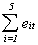1  13.9078   0.0017   8.3588  ş0.9610   0.9828   22.2902   496.8539
2   5.4162   0.0847   9.2193   4.0984  ş3.4472   15.3716   236.2871
3  ş2.2379 ş22.9662 ş21.9916 ş34.5861   3.5393  ş78.2425  6121.896
4   5.2731   6.7375   2.4383   9.1345  ş5.0507   18.5328   343.4657
5   3.2350  ş8.4987 ş16.2592  ş9.8896   5.2747  ş26.1378   683.1872
6   5.6584  ş5.7907   5.7102  16.9828  ş7.4779   15.0828   227.4911
7  16.4835  ş3.3493  11.6723  23.2280 ş10.4094   37.6250  1415.641
8   3.3982   4.8737   8.0163  ş8.9838  ş9.3291   ş2.0245     4.0986
9   0.4236 ş11.6166  ş5.6723   0.2788  ş1.0133  ş17.5997   309.7504
10  11.7964   3.2405  ş4.9625   6.9533  ş1.9256   15.1020   228.0710 10066.74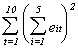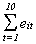63.3545 ş37.2832  ş3.47025  6.2554 ş28.8565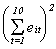4013.795 1390.038 12.04263 39.13009 832.7027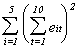6287.709